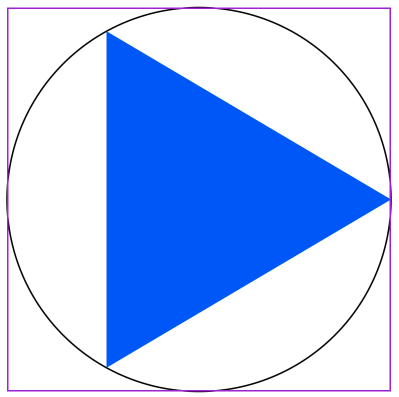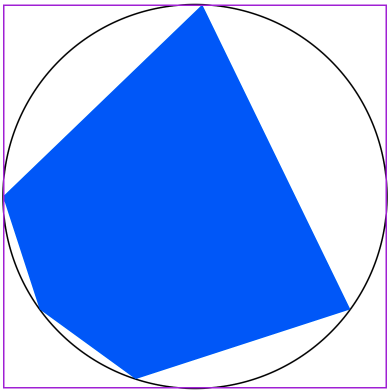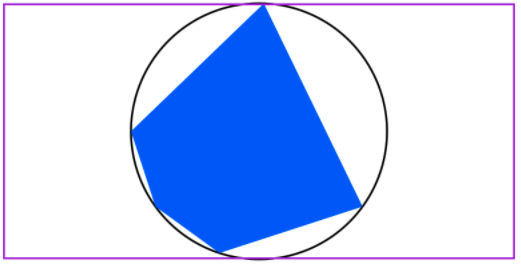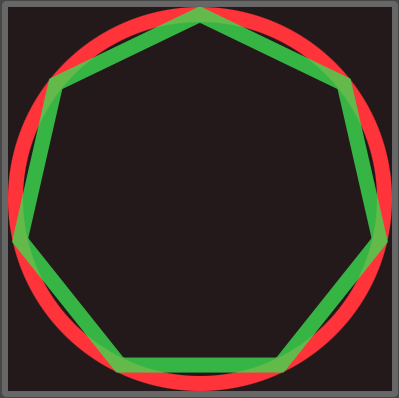# Swiftpack.co - nutterfi/Shapes as Swift Package

Swiftpack.co is a collection of thousands of indexed Swift packages. Search packages.
nutterfi/Shapes 0.2.17
A Swift package to expand the library of usable shapes in SwiftUI projects
⭐️ 8
🕓 1 week ago
iOS macOS watchOS tvOS
.package(url: "https://github.com/nutterfi/Shapes.git", from: "0.2.17")

# Shapes

This Swift package can be used to easily build SwiftUI shapes that conform to the `Shape` protocol. Most of the well-known shapes are in here, and some not-so-well-known shapes are here too!

## Requirements

Shapes requires a minimum of macOS 11, iOS 14, and tvOS 14.

## Under construction

### NFiShape

Most of the types that are defined in this project are structs that adhere to SwiftUI's `Shape` protocol. Because `Shape` is already taken, I settled on `NFiShape` as a base protocol that all shapes must conform to.

``````public protocol NFiShape: InsettableShape {
var inset: CGFloat { get set }
}
``````

The definition shows that `NFiShape` conforms to `InsettableShape`, which allows us to use insets on our shapes. ;)

One of the great things about defining `NFiShape` as a protocol is that we can implement a protocol extension that covers our bases as it relates to `InsettableShape`:

``````public extension NFiShape {
func inset(by amount: CGFloat) -> some InsettableShape {
var me = self
me.inset += amount
return me
}
}
``````

Now any shape that in turn conforms to `NFiShape` will automatically get this behavior for free.

### Polygon

A `Polygon` is a closed shape that consists of multiple line segments. It is defined in this project as a protocol:

``````/// A plane figure that is described by a finite number of straight line segments connected to form a closed polygonal chain
public protocol Polygon: NFiShape {
var sides: Int { get }
func vertices(in rect: CGRect) -> [CGPoint]
}
``````

Each `Polygon` is defined by the number of sides. In order for the shape to be drawn, the `vertices` of each polygon are also required. These are managed via a `CGPoint` array. The vertices are obtained with a method in order to determine the position given a `CGRect`

Not all shapes in this library will conform to `Polygon` because they may include curved surfaces. However, all shapes should conform to `NFiShape` to take advantage of the insettable feature.

#### Polygon Example: SimplePolygon

The simplest Polygon example in the library is appropriately named `SimplePolygon`. Provide an array of ratios in the initializer to construct a polygon with points around the unit circle that are contained in the shape's frame.

For example, if one were to define a polygon with points at 0.33, 0.67, and 1:

``````SimplePolygon(ratios: [0.33, 0.67, 1])
``````

this tells the library to generate a polygon with vertices at 33%, 67%, and 100% distance traveled around the unit circle. (0% starts at the point facing east in the following illustration)The code used to generate this diagram is

``````struct SimplePolygon_Previews: PreviewProvider {
static var previews: some View {
ZStack {
Circle().stroke()  // the unit circle
SimplePolygon(ratios: [0.33, 0.67, 1])
.foregroundColor(Color.blue)
}
.border(Color.purple)
}
}
``````

You can provide as many ratios as desired, in any order, and the shape will render them appropriately:

``````struct SimplePolygon2_Previews: PreviewProvider {
static var previews: some View {
ZStack {
Circle().stroke() // the unit circle
SimplePolygon(ratios: [0.1, 0.5, 0.756, 0.3, 0.4])
.foregroundColor(Color.blue)
}
.border(Color.purple)
}
}
``````NOTE: For non-square frames, the polygon will be rendered in the center of the frame. The unit circle is measured based on the smallest dimension of the `CGRect`:

``````struct SimplePolygon3_Previews: PreviewProvider {
static var previews: some View {
ZStack {
Circle().stroke()
SimplePolygon(ratios: [0.1, 0.5, 0.756, 0.3, 0.4])
.foregroundColor(Color.blue)
}
.border(Color.purple)
.frame(width: 256, height: 128)
}
}
``````### Polygon Example: ConvexPolygon

A convex polygon, also known as a Regular Polygon, is a polygon whose sides are all equal length and whose vertices are equally distributed around the unit circle.

`ConvexPolygon` is defined as a struct that conforms to the `RegularPolygon` protocol. As a protocol, `RegularPolygon` requires the following properties and methods:

``````/// A polygon with equal-length sides and equally-spaced vertices along a circumscribed circle
public protocol RegularPolygon: Polygon {}
``````

That's right, it's empty! `RegularPolygon` conforms to `Polygon` without any additional requirements. The reason that `RegularPolygon` exists is to implement the `vertices(in: CGRect)` method via a protocol extension:

``````public extension RegularPolygon {

/// obtains the equally spaced points of a polygon around a circle inscribed in rect, arranged clockwise starting at the top of the unit circle
func vertices(in rect: CGRect) -> [CGPoint] {
vertices(in: rect, offset: .zero)
}

/// obtains the equally spaced points of a polygon around a circle inscribed in rect, arranged clockwise starting at the top of the unit circle, with any additional rotational offset
func vertices(in rect: CGRect, offset: Angle = .zero) -> [CGPoint] {
let r = min(rect.size.width, rect.size.height) / 2
let origin = CGPoint(x: rect.midX, y: rect.midY)
let array: [CGPoint] = Array(0 ..< sides).map {
let theta = 2 * .pi * CGFloat(\$0) / CGFloat(sides) + CGFloat(offset.radians) - CGFloat.pi / 2  // pointing north!
return CGPoint(x: origin.x + r * cos(theta), y: origin.y + r * sin(theta))
}
return array
}
}
``````

In the code above, I made the conscious choice to rotate the origin of the unit circle to be facing up. Most polygon drawings I've seen in textbooks and the internet (no citation provided, sorry) show a corner pointing to the top of the image, so that's the convention that is used here.

The second method, `vertices(in: CGRect, offset: Angle)` allows the user to specify additional rotational offset as desired.

These methods are useful to obtain the vertices for other shapes that are not polygons as well, e.g. `ReuleauxPolygon` and `Torx` with their curved sides.

Now that we understand how `RegularPolygon` is used, it's time to learn about `ConvexPolygon`. The initializer takes a number of sides, like so:

``````ConvexPolygon(sides: 7)
``````

This shape can be illustrated with the following example:

``````struct ConvexPolygon_Previews: PreviewProvider {
static var previews: some View {
ZStack {
Circle() // unit circle
.strokeBorder(Color.red, lineWidth: 10)

ConvexPolygon(sides: 7)
.strokeBorder(Color.green.opacity(0.8), lineWidth: 10)
}
}
}
``````### GitHub

 link Stars: 8 Last commit: 1 week agoSomething's broken? Yell at me @ptrpavlik. Praise and feedback (and money) is also welcome.

### Release Notes

First Public Beta Release
2 years ago

First Public Beta Release

Swiftpack is being maintained by Petr Pavlik | @ptrpavlik | @swiftpackco | API | Analytics